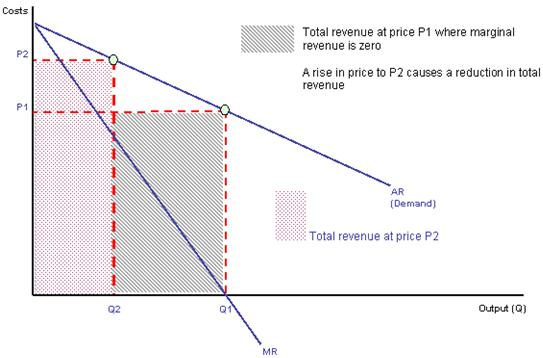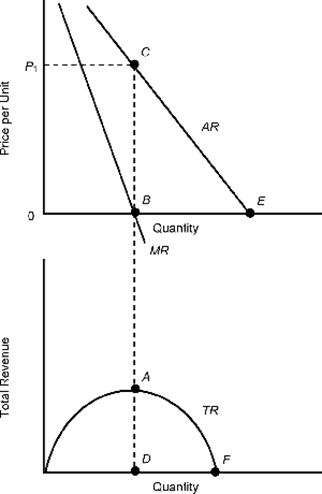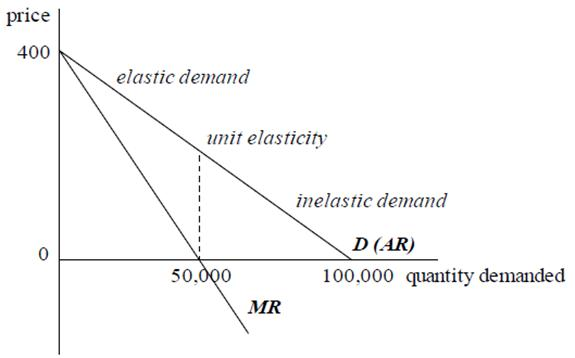# Revenues

## Revenues

### Meaning of revenue

Revenue is the amount or money received from the sale of output to the consumer.

### Total, Average and Marginal revenue

Total revenue is the total amount received for the sale of goods and services. When goods are sold at a single price, the total revenue is price × quantity. Thus total revenue is calculated as price times the quantity sold As depicted in the diagram below Total revenue is the area under the demand curveAs in the figure above people will buy Q1 units of the good at the price P1and thus Total Revenue will be P1 x Q1. The area of the rectangle below the demand curve is nothing but P1 X Q1 which is the height and the width respectively.
Marginal revenue is the increase in total revenue caused by an increase in output of one unit.
Mathematically, marginal revenue can be defined as:
d(total revenue)/d(output)
Average revenue (AR) is defined as total revenue divided by the output level. This is the price charged per unit.
AR = TR/ Qd
Now we also know that TR = P X Qd
Solving both the equations we can conclude that AR = P. Since price is the average revenue, then the demand curve is the average revenue curve
The table below shows the quantity demanded for a product at various prices (average revenue) and also the total and marginal revenue

 Price per unit (average revenue) \$ Quantity Demanded (Qd) Units Total Revenue (TR) \$ Marginal Revenue (MR) \$ 400 220 88,000 - 370 340 125,800 315 340 460 156,400 255 310 580 179,800 195 280 700 196,000 135 250 820 205,000 75 220 940 206,800 15 190 1060 201,400 -45

This pattern of marginal revenue falling faster than price at all levels of output, and eventually becoming negative, is quite typical
Marginal revenue falls for two reasons:

1. As output increases, the selling price must fall (since demand curves usually slope downwards)
2. To sell an extra unit, the price charged on all previous units of output must usually be reduced.

In general, marginal revenue = additional revenue earned from selling last unit alone – revenue lost by selling existing output at a lower price

### Relationship between total, average and marginal revenue

In the table above, as price per unit of the good decreases, demand increases thereby increasing total revenue. Since average revenue falls as more units are sold, marginal revenue declines and ultimately MR becomes negative which leads to the fall in total revenue.
To summarise:

• When AR (Price) starts falling MR also falls
• When MR is zero, TR is maximum
• When MR becomes negative, TR starts decreasing

These relationships are also depicted in the figure below:Notice that when the demand curve is a straight line, the MR curve is always twice as steep as the AR curve, so it cuts the x-axis halfway between 0 and the point where the demand curve cuts the axis.

### Elasticity of demand and total revenue

Elasticity falls as price falls/output increases. As shown in the figure below when demand is elastic, a fall in price (i.e. an increase in output) will increase revenue. So, when demand is elastic, marginal revenue is positive. Similarly, when demand is inelastic, marginal revenue is negative. When demand has unit elasticity, marginal revenue is zero.The usual pattern for a marginal revenue curve is to slope down at all output levels, and to do so more steeply than the demand curve. Marginal revenue becomes negative when demand becomes inelastic.

Microeconomics | Microeconomics Help | Revenues | Microeconomics Costs | Revenue | Net Revenue | Internal Revenue Service | What Is Revenue | Sales Revenue | Department Of Revenue | Online tutoring